## (TD) 4.4 State Label Equation Alternative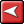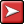## (TD) 4.4 State Label Equation AlternativeAnother way to generate analog signals is to use Waveform and Label equations. This is a little simpler than writing the Python code required when using the Waveform Equation Blocks of the previous section. However, once the waveform is generated you cannot edit it using the original equation. You will have to either edit each edge, or delete a section of the waveform and regenerate it.

In this example, we will use a Waveform Equation (not a block equation) to generate the blank waveform segments. Then use a State Label equation to insert the values onto the segments. We will be inserting an extra segment for each value change so that we can use it in the next section to render a "stepped" waveform even though we have not checked the Use Straight Edges checkbox.

Use a Waveform Equation to Generate the Valid segments:

 •Add a new signal and double click to open the Signals Properties dialog. Name the signal SIG0_Digital and set the radix to real.•Notice the default equation in the Wfm Eqn box. It is an example of all the syntax accepted by the generator. Each segment is represented by a time=state pair. If you forget the syntax just generate this equation and figure it out from the waveform.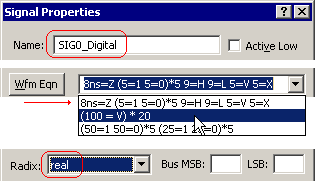•Enter (50=V)*5 to generate 5 valid segments that are 50ns long.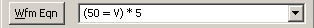•Press the Wfm Eqn to generate the waveform segments. Leave the dialog open.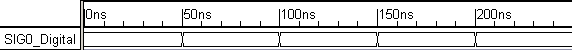Use a simple Label Equation to label the segments:

The Label Equation box accepts equations that generate state values for the signal's waveform segments. This example demonstrates the concatenation operator (the comma). The concatenation operator can also be combined with the decrement and increment label equations to create more complex patterns.

 •In the Label Eqn box, type 1.5, 1.5, 4.5, 4.5, 0. •Press the Label Eqn button to apply the equation.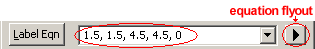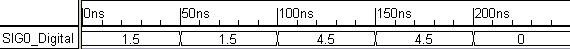Create an Analog Signal:

 •Copy and Paste SIG0_Digital by selecting the signal name and using the Ctrl-C and Ctrl-V keys. Double click on the new signal to open the Signal Properties dialog.
 •Name the signal SIG0_Analog•Check the Analog Display check box. •Set the Size to 2, to expand the waveform.•Press the Apply button to apply the changes to the signal.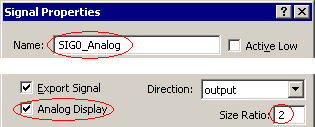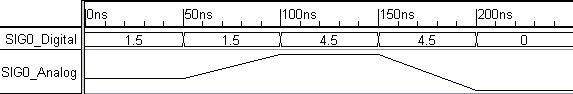Although the above analog signal appears to have ramps in it, it will look like a stepped waveform when exported to a digital simulator. To create ramps that export to a digital simulator, use the Ramp label equation as discussed later in this tutorial.